Like   Tweet   Pin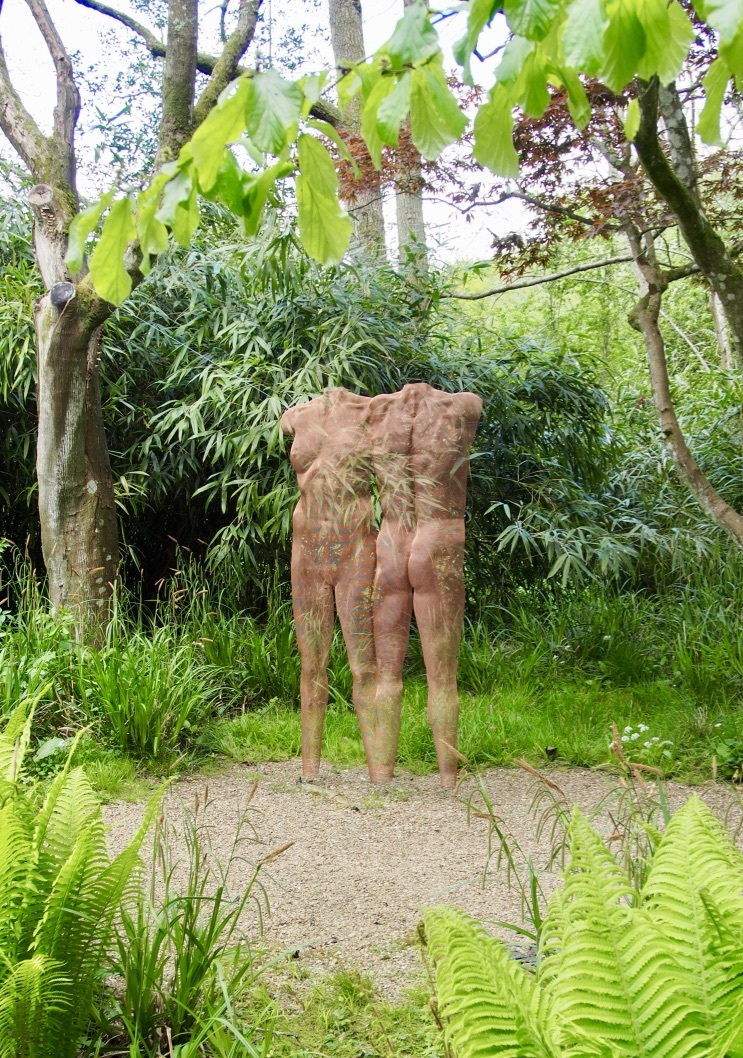Sculpture ’ICONII’, bronzemesh
 /* styles */ Dear all, the U.K. summer is still ongoing as is the fabulous outdoor exhibition at

## Hannah Peschar Sculpture Garden, Surrey

 table div table+table+table+table+table div table{width:100%;padding:0}table div table+table+table+table+table div table table{padding:0;float:left!important;width:45.094%!important}table div table+table+table+table+table div table table+table td,table div table+table+table+table+table div table td{padding-left:0;padding-right:0}table div table+table+table+table+table div table table td{padding-left:0;padding-right:20px}table div table+table+table+table+table div table table+table{float:left!important;width:54.906%!important}/* styles */
 /* styles */ Until 27th October: Sculpture ’ICONII’, ’VENUS’ as well as ’SKIN I/SKIN II’ are on show – many thanks to Vikki and Nichola for great photos of the installations at the Hannah Peschar Sculpture Garden, curated by Anthony Paul and Vikki Leedham. The Hannah Peschar Sculpture Garden, Standon Lane, Dorking RH5 5QU Tel. 01306 627269
 table div table+table+table+table+table+table+table div table{width:100%;padding:0}table div table+table+table+table+table+table+table div table img{width:96.23%;padding:0;float:none}table div table+table+table+table+table+table+table div table td{width:100%;padding:0 1.88% 18px}/* styles */## New Sculpture Videos Online:

 table div table+table+table+table+table+table+table+table+table div table td,table.module-8{width:100%;padding:0}table div table+table+table+table+table+table+table+table+table div table{width:100%;float:none;margin-left:auto;margin-right:auto;padding:0}table div table+table+table+table+table+table+table+table+table div table a{border:0 none;text-decoration:none}table div table+table+table+table+table+table+table+table+table div table img{width:100%!important;border:0 none;text-decoration:none}/* styles */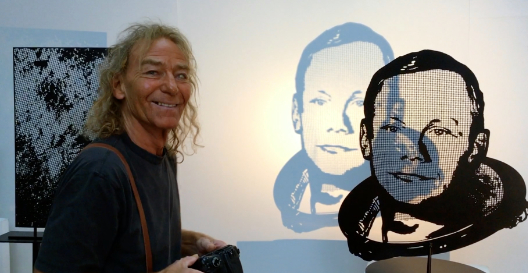Video: sculpture ’NEIL ARMSTRONG’ and ’FOOTPRINT’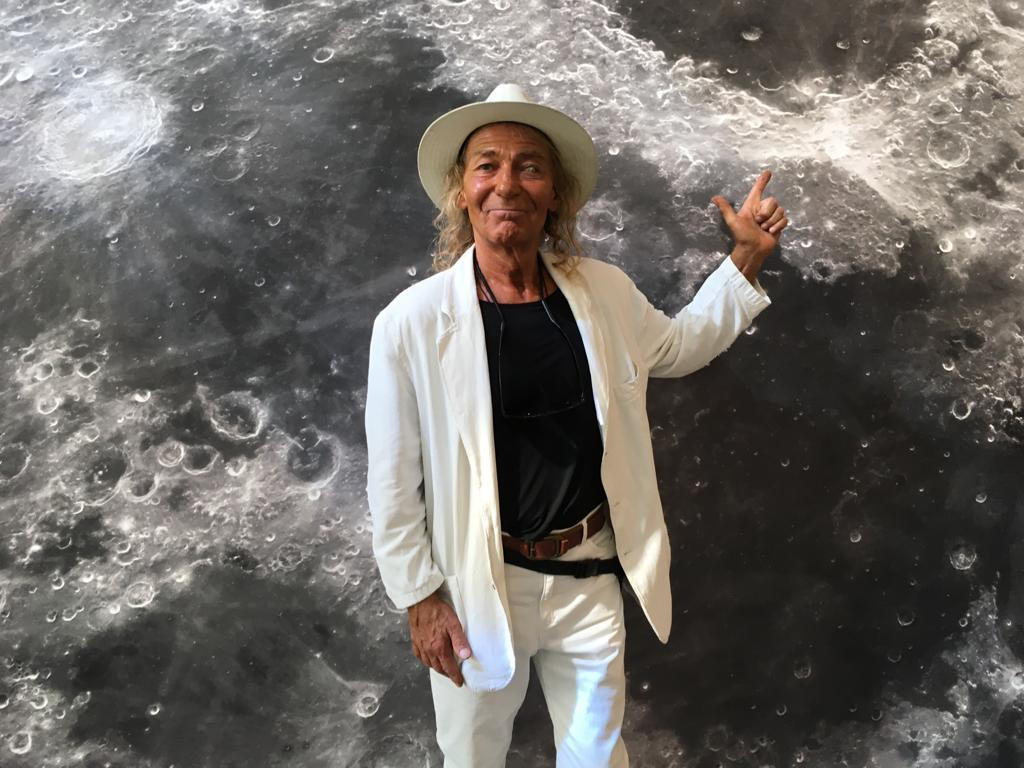The combination of photography and steel panel proves to be able to realise extraordinary results - these recently commissioned sculptures ’SPACEMAN’, ’NEIL ARMSTRONG’ and ’FOOTPRINT’ celebrate the 50th anniversary of the most famous footprint ’in the world’ from Neil Armstrong - which is on the moon!

Many thanks to Jan Pulsford for the superb music featuring in my videos!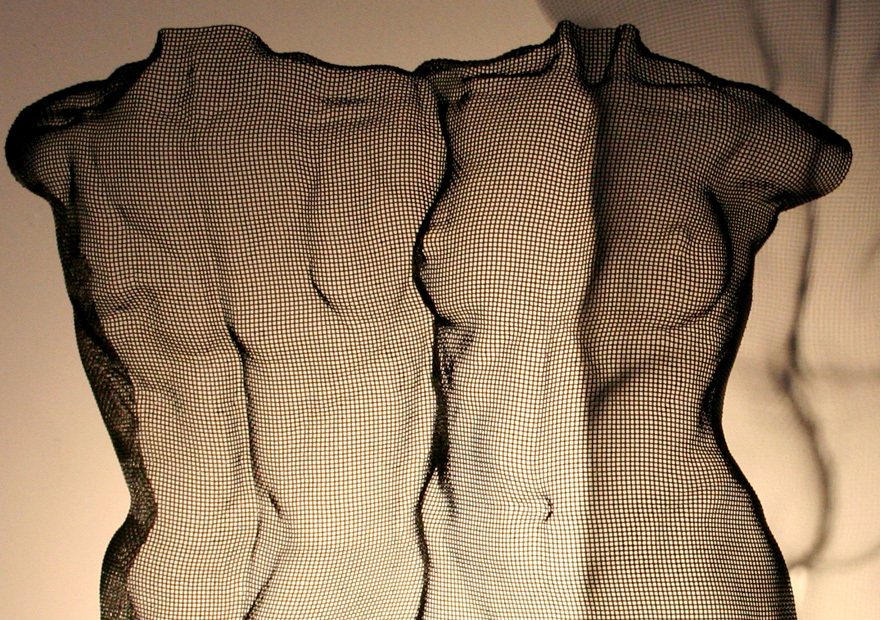This short video presents sculpture ’PNUU’ 2019
 table div table+table+table+table+table+table+table+table+table+table+table+table+table div table{width:100%;padding:0}table div table+table+table+table+table+table+table+table+table+table+table+table+table div table img{width:96.23%;padding:0;float:none}table div table+table+table+table+table+table+table+table+table+table+table+table+table div table td{width:100%;padding:0 1.88% 18px}/* styles */## Contini Contemporary, London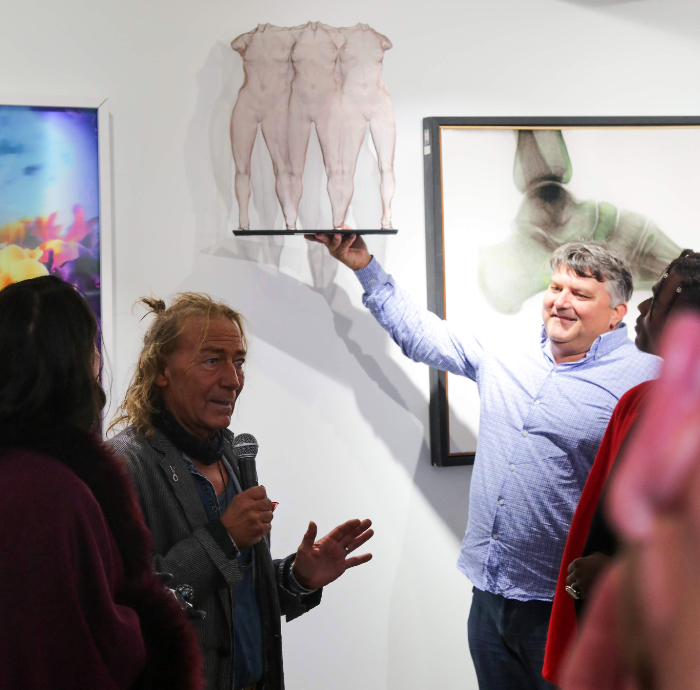Teamwork from David and Fulvio's pop-up sculpture show!
 /* styles */ It's been an exciting summer too for Contini Contemporary who have staged a series of mega impressive events, exhibitions and installations including artist Tony Cragg at the Uffizzi in Florence, Italy, and a succession of artist ENDLESS street art and other events and performances initiated and promoted by Cristian Contini. Most recently they have collaborated in creating a liaison between fashion and art in London. Many thanks to Fulvio Granocchia for giving me a microphone and a pop-up show during last week's fashion event "Travel in Style" at their Mayfair art gallery. In the picture: sculpture ’ISIUU’ 2019, a triple figure compostion in wiremesh. Here's a short video of this sculpture with finishing touches at the studio.
 table div table+table+table+table+table+table+table+table+table+table+table+table+table+table+table+table+table div table{width:100%;padding:0}table div table+table+table+table+table+table+table+table+table+table+table+table+table+table+table+table+table div table img{width:96.23%;padding:0;float:none}table div table+table+table+table+table+table+table+table+table+table+table+table+table+table+table+table+table div table td{width:100%;padding:0 1.88% 18px}/* styles */## Bel Air Fine Art Galleries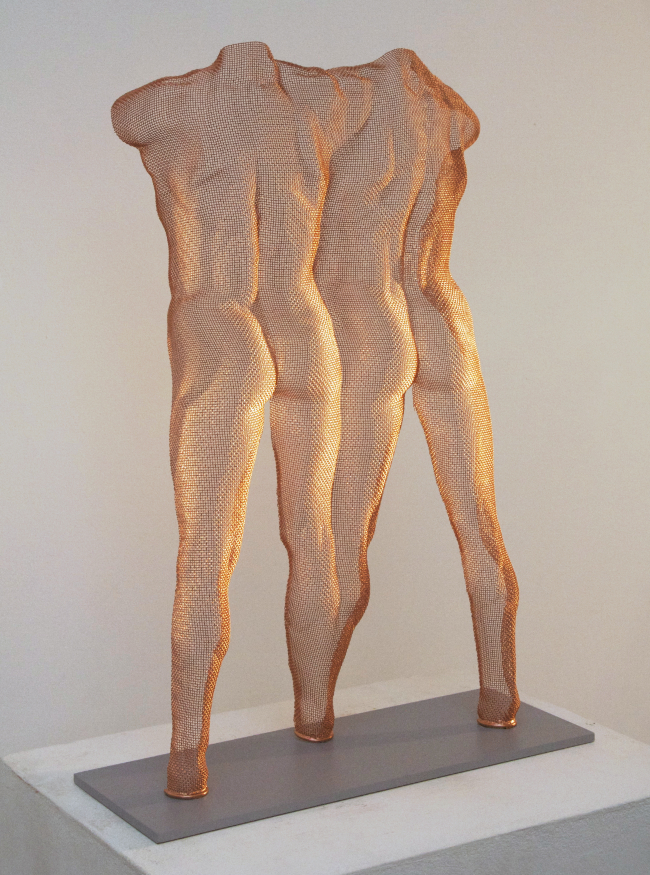Scupture composition ’VENAS’ at Bel-Air Fine Art, London
 /* styles */ Frieze Artfair 2019 kicks off with the opening night on 2nd October and ends 6th October. Bel-Air Fine Art Galleries are exhibiting a selection of my work in London during this art event - as well as in Geneva, Crans-Montana & Gstaad, Switzerland, Knokke in Belgium and Paris Rivoli.
 table div table+table+table+table+table+table+table+table+table+table+table+table+table+table+table+table+table+table+table+table+table div table{width:100%;padding:0}table div table+table+table+table+table+table+table+table+table+table+table+table+table+table+table+table+table+table+table+table+table div table img{width:96.23%;padding:0;float:none}table div table+table+table+table+table+table+table+table+table+table+table+table+table+table+table+table+table+table+table+table+table div table td{width:100%;padding:0 1.88% 18px}/* styles */## Watson Gallery, Edinburgh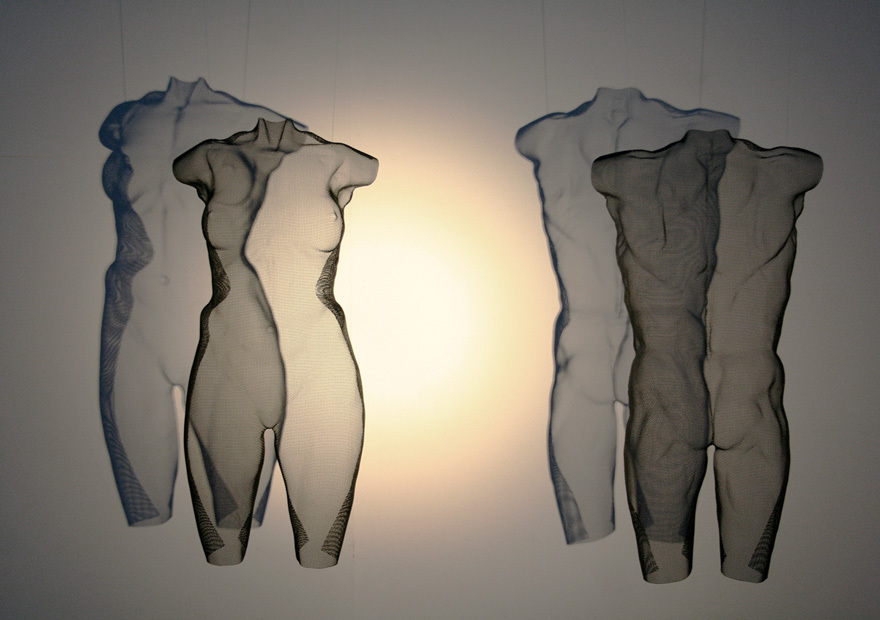Torso Sculpture ‘CENS‘ and ‘CENZ‘ at the Watson Gallery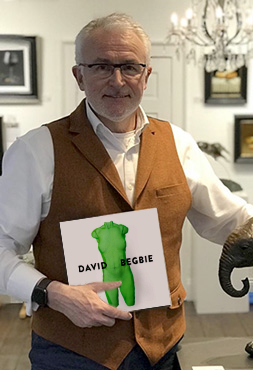Bob Corsie, Watson Gallery, Edinburgh
 table div table+table+table+table+table+table+table+table+table+table+table+table+table+table+table+table+table+table+table+table+table+table+table+table+table div table,table.module-24{width:37.74%;float:left;padding:0}table div table+table+table+table+table+table+table+table+table+table+table+table+table+table+table+table+table+table+table+table+table+table+table+table+table div table a{border:0 none;text-decoration:none}table div table+table+table+table+table+table+table+table+table+table+table+table+table+table+table+table+table+table+table+table+table+table+table+table+table div table img{width:100%!important;border:0 none;text-decoration:none}table div table+table+table+table+table+table+table+table+table+table+table+table+table+table+table+table+table+table+table+table+table+table+table+table+table div table td{width:100%;padding:0 20px 20px 0}/* styles */ I am delighted to now be showing at Watson Gallery in my birthtown Edinburgh which is also home to United Wire Ltd. who have supplied me with steel and bronzemesh since my student days. Watson Gallery, 39 Queen Street, Edinburgh EH2 3NH. Tel: 0131 225 7053 Opening times Mon-Sat 11am–6:pm, Sun 12–5 pm.
 table div table+table+table+table+table+table+table+table+table+table+table+table+table+table+table+table+table+table+table+table+table+table+table+table+table+table div table{width:100%;padding:0}table div table+table+table+table+table+table+table+table+table+table+table+table+table+table+table+table+table+table+table+table+table+table+table+table+table+table div table img{width:96.23%;padding:0;float:none}table div table+table+table+table+table+table+table+table+table+table+table+table+table+table+table+table+table+table+table+table+table+table+table+table+table+table div table td{width:100%;padding:0 1.88% 18px}/* styles */## ‘Biennale Brabant‘ Tilburg, The Netherlands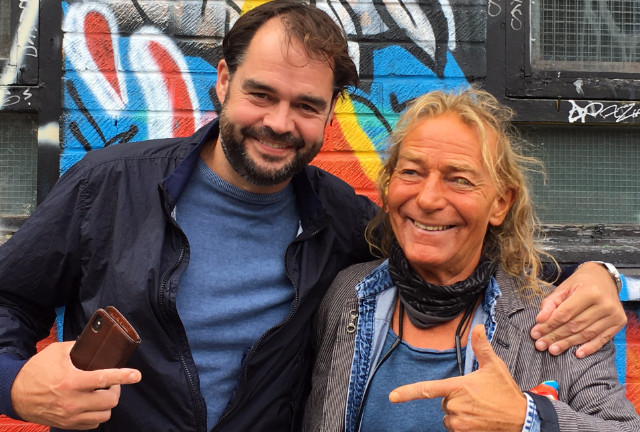Joris Van Loon and David Begbie in Shoreditch, London
 table div table+table+table+table+table+table+table+table+table+table+table+table+table+table+table+table+table+table+table+table+table+table+table+table+table+table+table+table+table div table,table.module-28{width:40.94%;float:left;padding:0}table div table+table+table+table+table+table+table+table+table+table+table+table+table+table+table+table+table+table+table+table+table+table+table+table+table+table+table+table+table div table a{border:0 none;text-decoration:none}table div table+table+table+table+table+table+table+table+table+table+table+table+table+table+table+table+table+table+table+table+table+table+table+table+table+table+table+table+table div table img{width:100%!important;border:0 none;text-decoration:none}table div table+table+table+table+table+table+table+table+table+table+table+table+table+table+table+table+table+table+table+table+table+table+table+table+table+table+table+table+table div table td{width:100%;padding:0 20px 20px 0}/* styles */ I've worked with Joris van Loon for nearly 20 years and I'm pleased to take part in the next event: 8 to 24 November 2019: Van Loon Galleries is staging the next edition of the Biennale Brabant, central Holland (NS-Plein, Koepelhal, Tilburg), a unique International art exhibition not to be missed. Get tickets online: Van Loon Galleries I will be exhibiting a number of sculptures including a new work based on theme "A Walk in the Park" which has been chosen as a general theme for this year's event.
 table div table+table+table+table+table+table+table+table+table+table+table+table+table+table+table+table+table+table+table+table+table+table+table+table+table+table+table+table+table+table div table{width:100%;padding:0}table div table+table+table+table+table+table+table+table+table+table+table+table+table+table+table+table+table+table+table+table+table+table+table+table+table+table+table+table+table+table div table img{width:96.23%;padding:0;float:none}table div table+table+table+table+table+table+table+table+table+table+table+table+table+table+table+table+table+table+table+table+table+table+table+table+table+table+table+table+table+table div table td{width:100%;padding:0 1.88% 18px}/* styles */## Vecchiato Arte, Padova and Pietrasanta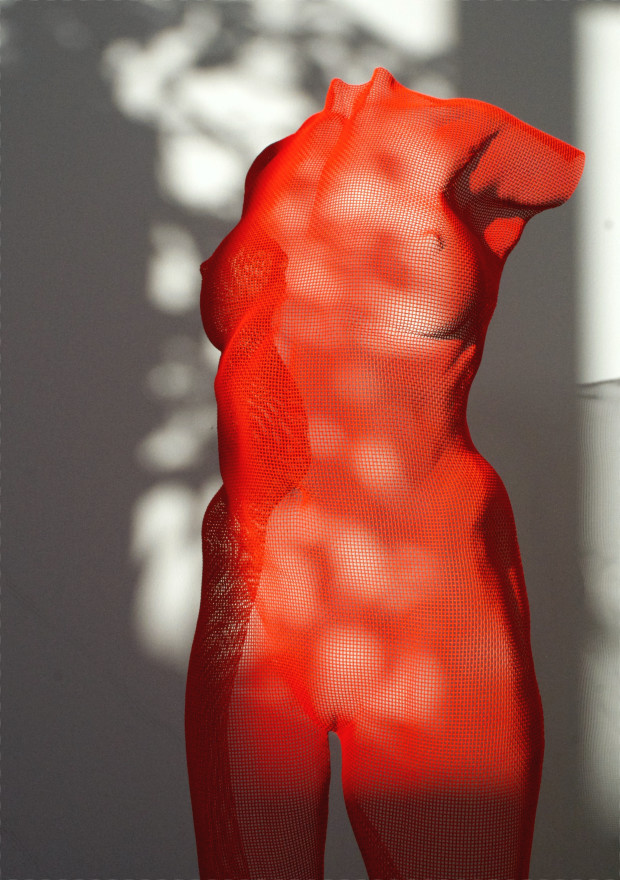‘FEME‘ sculpture in bright red finish
 /* styles */ This summer it's 12 years since I've first exhibited with Cinzia Vecchiato at Vecchiato Arte, Padova, Italy, during which time my sculpture has been presented with three solo shows and included in many group exhibitions and art fairs in Pietrasanta, Padova, Lucca, Bergamo, Milan and Bologna. I'm pleased to be represented by one of Italy's oldest established family galleries.
 table div table+table+table+table+table+table+table+table+table+table+table+table+table+table+table+table+table+table+table+table+table+table+table+table+table+table+table+table+table+table+table+table+table+table div table{width:100%;padding:0}table div table+table+table+table+table+table+table+table+table+table+table+table+table+table+table+table+table+table+table+table+table+table+table+table+table+table+table+table+table+table+table+table+table+table div table img{width:96.23%;padding:0;float:none}table div table+table+table+table+table+table+table+table+table+table+table+table+table+table+table+table+table+table+table+table+table+table+table+table+table+table+table+table+table+table+table+table+table+table div table td{width:100%;padding:0 1.88% 18px}/* styles */## Rarity Gallery, Mykonos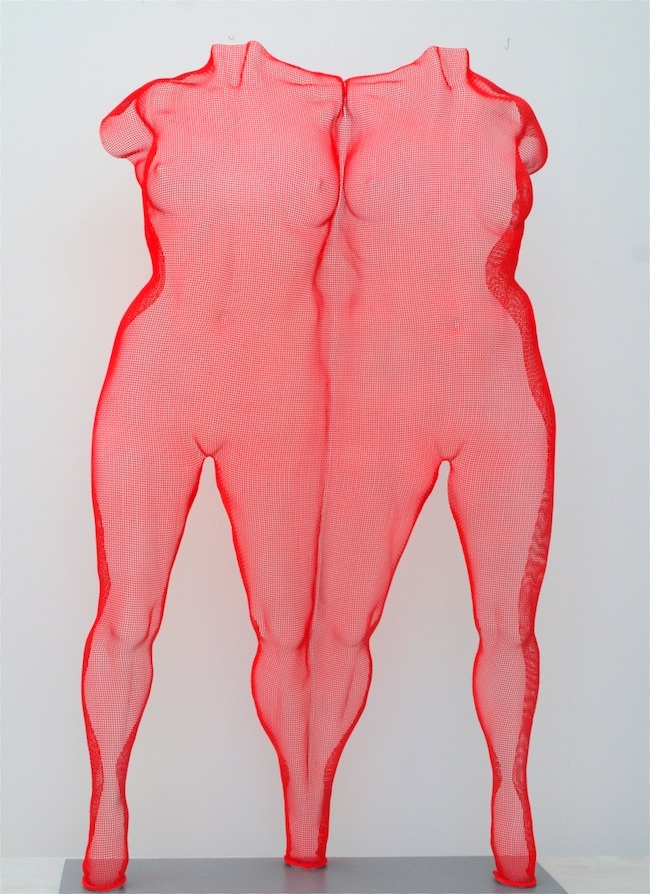‘FEMERGE‘, a steelmesh sculpture in bright red colour finish.
 /* styles */ It's been a long hot summer also in Greece. Rarity Gallery exhibiting a colourful selection of my steelmesh sculptures in their summer salon exhibitions over the last 5 years!
 table div table+table+table+table+table+table+table+table+table+table+table+table+table+table+table+table+table+table+table+table+table+table+table+table+table+table+table+table+table+table+table+table+table+table+table+table+table+table div table{width:100%;padding:0}table div table+table+table+table+table+table+table+table+table+table+table+table+table+table+table+table+table+table+table+table+table+table+table+table+table+table+table+table+table+table+table+table+table+table+table+table+table+table div table img{width:96.23%;padding:0;float:none}table div table+table+table+table+table+table+table+table+table+table+table+table+table+table+table+table+table+table+table+table+table+table+table+table+table+table+table+table+table+table+table+table+table+table+table+table+table+table div table td{width:100%;padding:0 1.88% 18px}/* styles */## Ruth Borchard Self-Portrait Prize Exhibition, London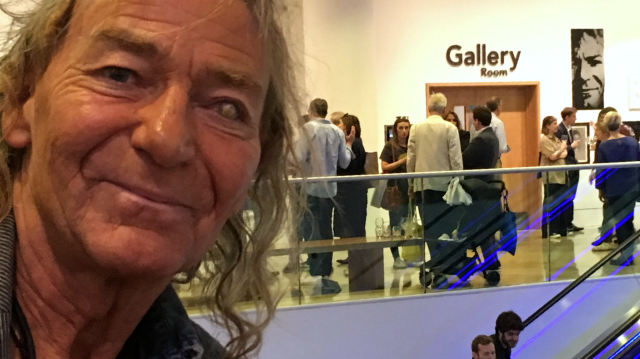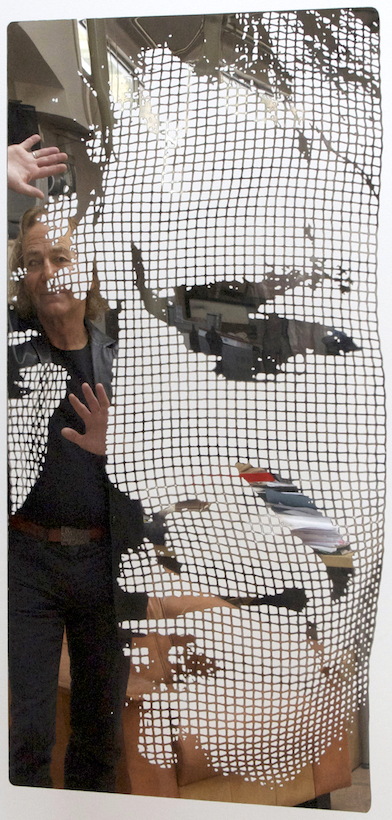David Begbie ‘Self-Portrait‘, semi-transparent steel

Free exhibition until 11th October:
The Ruth Borchard Self-Portrait Prize Exhibition currently showing at the public mezzanine of Kings Place, King's Cross, London, includes my self portrait.
Kings Place is situated just a few minutes’ walk from King’s Cross and St Pancras stations, London N1 9AG.

For this sculpture I have used my own technique of modelling in steelmesh and incorporating photography, using an industrial stainless steel sheet.

 table div table+table+table+table+table+table+table+table+table+table+table+table+table+table+table+table+table+table+table+table+table+table+table+table+table+table+table+table+table+table+table+table+table+table+table+table+table+table+table+table+table+table div table{width:100%;padding:0}table div table+table+table+table+table+table+table+table+table+table+table+table+table+table+table+table+table+table+table+table+table+table+table+table+table+table+table+table+table+table+table+table+table+table+table+table+table+table+table+table+table+table div table img{width:96.23%;padding:0;float:none}table div table+table+table+table+table+table+table+table+table+table+table+table+table+table+table+table+table+table+table+table+table+table+table+table+table+table+table+table+table+table+table+table+table+table+table+table+table+table+table+table+table+table div table td{width:100%;padding:0 1.88% 18px}/* styles */## XII ‘Veleziana‘, Italy 18-20 October

 table div table+table+table+table+table+table+table+table+table+table+table+table+table+table+table+table+table+table+table+table+table+table+table+table+table+table+table+table+table+table+table+table+table+table+table+table+table+table+table+table+table+table+table+table div table td,table.module-43{width:100%;padding:0}table div table+table+table+table+table+table+table+table+table+table+table+table+table+table+table+table+table+table+table+table+table+table+table+table+table+table+table+table+table+table+table+table+table+table+table+table+table+table+table+table+table+table+table+table div table{width:100%;float:none;margin-left:auto;margin-right:auto;padding:0}table div table+table+table+table+table+table+table+table+table+table+table+table+table+table+table+table+table+table+table+table+table+table+table+table+table+table+table+table+table+table+table+table+table+table+table+table+table+table+table+table+table+table+table+table div table a{border:0 none;text-decoration:none}table div table+table+table+table+table+table+table+table+table+table+table+table+table+table+table+table+table+table+table+table+table+table+table+table+table+table+table+table+table+table+table+table+table+table+table+table+table+table+table+table+table+table+table+table div table img{width:100%!important;border:0 none;text-decoration:none}/* styles */
 /* styles */ FineArtSails is an international project combining sailing, art, charity and celebrates the first keel boat, the Queen of dinghies: the Star. With its unique art sails painted by International artists FineArtSails will race again: From Trieste with the Star Class Adriatic fleet 11th October and at the Veleziana 19th October, starting 3pm at Piazza San Marco, Venice. On Sunday the Star Class will join the Veleziana Regatta. The race can be watched from ashore, for an unforgettable spectacle. More info: www.veleziana.com and www.compagniadellavela.org. Ma sail design ‘An Eye for the Buoys‘ is designed from sculpture ‘EVE‘ 2008, the image of my sail, is available as a steel panel sculpture in two sizes and three colours: black, red and mirror. Enquiries welcome!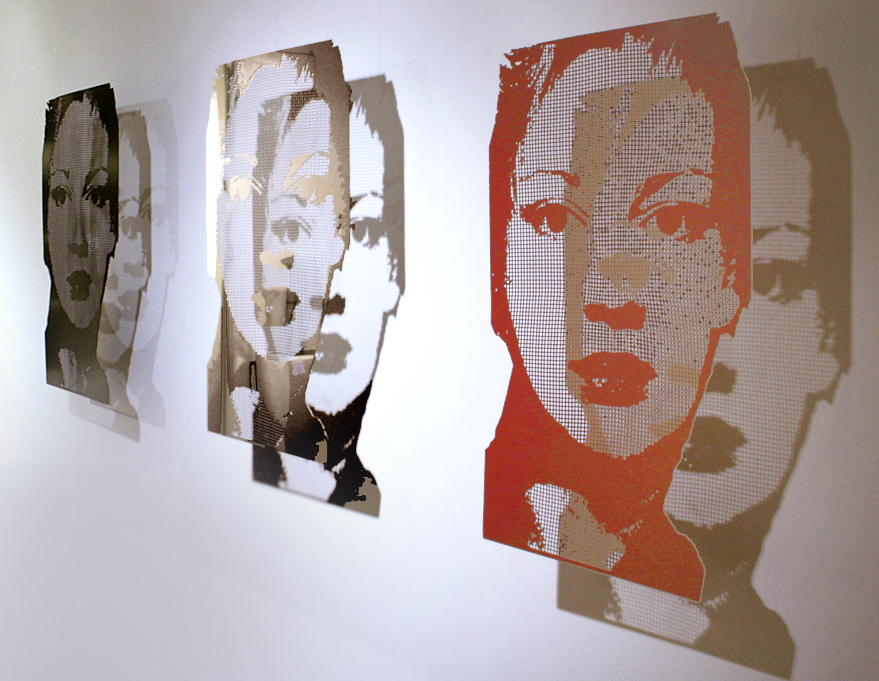‘EVE‘ 2008, steelpanel sculpture (suspended or mounted)
 table div table+table+table+table+table+table+table+table+table+table+table+table+table+table+table+table+table+table+table+table+table+table+table+table+table+table+table+table+table+table+table+table+table+table+table+table+table+table+table+table+table+table+table+table+table+table+table div table{width:100%;padding:0}table div table+table+table+table+table+table+table+table+table+table+table+table+table+table+table+table+table+table+table+table+table+table+table+table+table+table+table+table+table+table+table+table+table+table+table+table+table+table+table+table+table+table+table+table+table+table+table div table img{width:96.23%;padding:0;float:none}table div table+table+table+table+table+table+table+table+table+table+table+table+table+table+table+table+table+table+table+table+table+table+table+table+table+table+table+table+table+table+table+table+table+table+table+table+table+table+table+table+table+table+table+table+table+table+table div table td{width:100%;padding:0 1.88% 18px}/* styles */## 40 Savile Row, London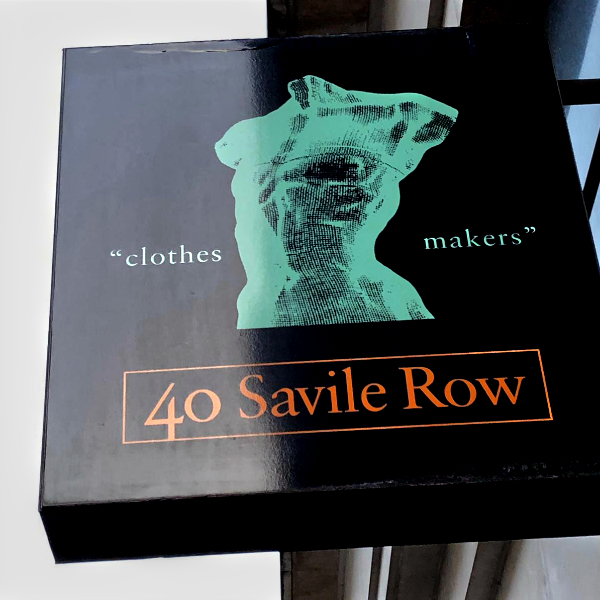40 Savile Row Logo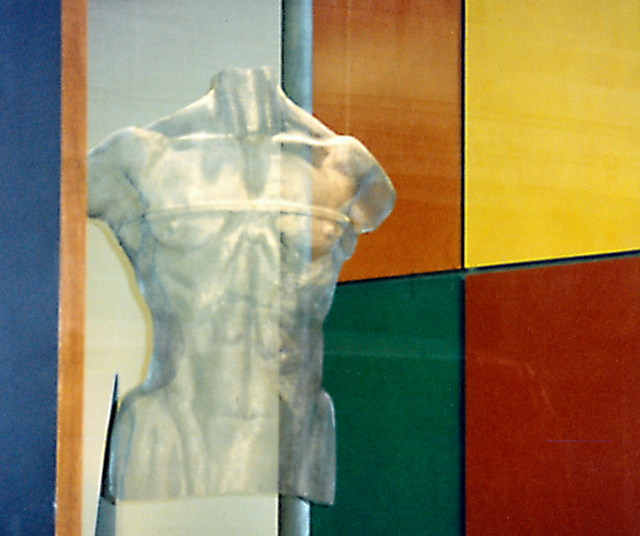’METRE’ sculpture
 /* styles */ Cristian Contini recently sent me this photo – nice to be greeted by my earlier sculpture ’METRE’ which seems to have a timeless appeal. Especially created for 40 Savile Row this image of brazen masculinity is adorned with a taylor's tape measure stretched across the chest. A trip down memory lane for me or more correctly Savile Row!
 table div table+table+table+table+table+table+table+table+table+table+table+table+table+table+table+table+table+table+table+table+table+table+table+table+table+table+table+table+table+table+table+table+table+table+table+table+table+table+table+table+table+table+table+table+table+table+table+table+table+table+table div table{width:100%;padding:0}table div table+table+table+table+table+table+table+table+table+table+table+table+table+table+table+table+table+table+table+table+table+table+table+table+table+table+table+table+table+table+table+table+table+table+table+table+table+table+table+table+table+table+table+table+table+table+table+table+table+table+table div table img{width:96.23%;padding:0;float:none}table div table+table+table+table+table+table+table+table+table+table+table+table+table+table+table+table+table+table+table+table+table+table+table+table+table+table+table+table+table+table+table+table+table+table+table+table+table+table+table+table+table+table+table+table+table+table+table+table+table+table+table div table td{width:100%;padding:0 1.88% 18px}/* styles */## Get an artwork at "cARTes Postale" Fundraiser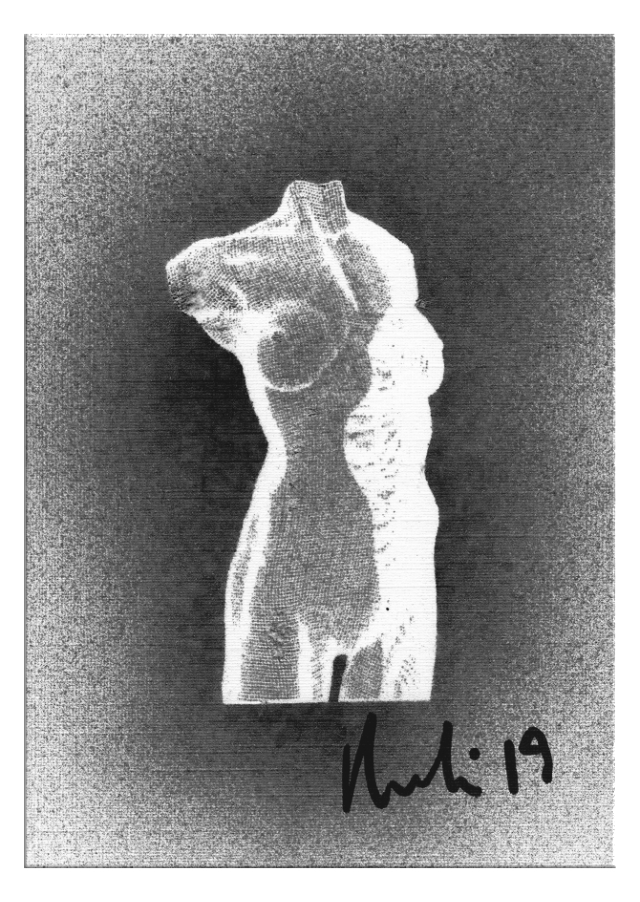Donation ‘STILL‘, mono-print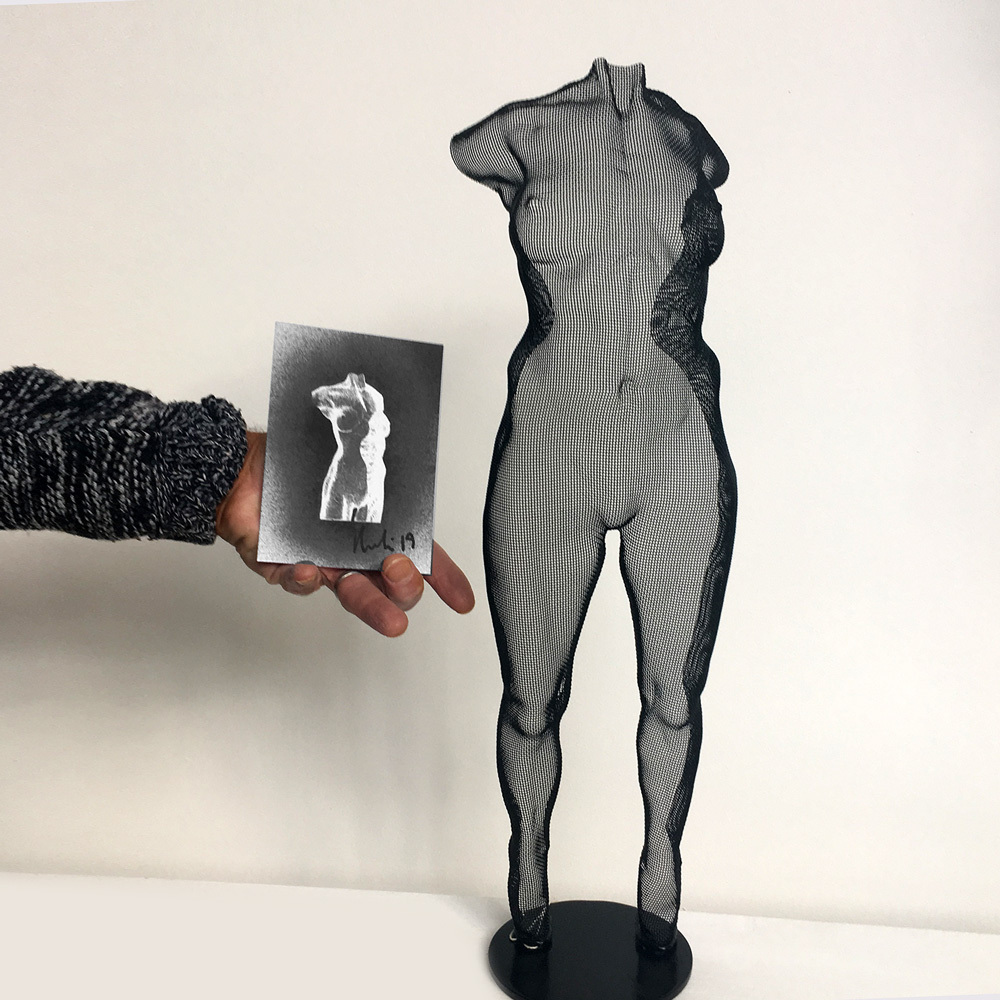Mono-print and unique steelmesh sculpture
 /* styles */ To support the Erlestoke Prison, the Salisbury Museum and Judy Squarey organised the fund raiser "cARTes Postale" including an auction in the evening on 25th October of ten art works, given by artists. Vouchers for these postcards, some designed by artists (Antony Gormley and others) are priced online at £40.00 each and every voucher owner is guaranteed to get a postcard but it's a matter of luck which card you will win! All info: "The Friends of Erlestoke Prison". Some of the postcards are displayed in the Museum and I am delighted that my donation is on show.
 table div table+table+table+table+table+table+table+table+table+table+table+table+table+table+table+table+table+table+table+table+table+table+table+table+table+table+table+table+table+table+table+table+table+table+table+table+table+table+table+table+table+table+table+table+table+table+table+table+table+table+table+table+table+table+table div table{width:100%;padding:0}table div table+table+table+table+table+table+table+table+table+table+table+table+table+table+table+table+table+table+table+table+table+table+table+table+table+table+table+table+table+table+table+table+table+table+table+table+table+table+table+table+table+table+table+table+table+table+table+table+table+table+table+table+table+table+table div table img{width:96.23%;padding:0;float:none}table div table+table+table+table+table+table+table+table+table+table+table+table+table+table+table+table+table+table+table+table+table+table+table+table+table+table+table+table+table+table+table+table+table+table+table+table+table+table+table+table+table+table+table+table+table+table+table+table+table+table+table+table+table+table+table div table td{width:100%;padding:0 1.88% 18px}/* styles */## Press

 table div table+table+table+table+table+table+table+table+table+table+table+table+table+table+table+table+table+table+table+table+table+table+table+table+table+table+table+table+table+table+table+table+table+table+table+table+table+table+table+table+table+table+table+table+table+table+table+table+table+table+table+table+table+table+table+table+table div table td,table.module-56{width:100%;padding:0}table div table+table+table+table+table+table+table+table+table+table+table+table+table+table+table+table+table+table+table+table+table+table+table+table+table+table+table+table+table+table+table+table+table+table+table+table+table+table+table+table+table+table+table+table+table+table+table+table+table+table+table+table+table+table+table+table+table div table{width:100%;float:none;margin-left:auto;margin-right:auto;padding:0}table div table+table+table+table+table+table+table+table+table+table+table+table+table+table+table+table+table+table+table+table+table+table+table+table+table+table+table+table+table+table+table+table+table+table+table+table+table+table+table+table+table+table+table+table+table+table+table+table+table+table+table+table+table+table+table+table+table div table a{border:0 none;text-decoration:none}table div table+table+table+table+table+table+table+table+table+table+table+table+table+table+table+table+table+table+table+table+table+table+table+table+table+table+table+table+table+table+table+table+table+table+table+table+table+table+table+table+table+table+table+table+table+table+table+table+table+table+table+table+table+table+table+table+table div table img{width:100%!important;border:0 none;text-decoration:none}/* styles */
 /* styles */ A short interview with designer Carole Annett in Country&Town House Magazine, October issue 2019, just published. What sparks design envy in you? Scale and proportion – getting it right. If you cold live in any building, what would it be? Apply House, Number One London, so I can be the first person in Hyde Park each morning. The most you've ever spent on an artwork is... Several thousand (I inherited the money and wanted to buy something meaningful). It was a wire sculpture of a man's torso by David Begbie. Which artist would you most like to sit next to at the bar? Tracey Emin – I'd love to hear her thoughts on Trump and Boris.
 table div table+table+table+table+table+table+table+table+table+table+table+table+table+table+table+table+table+table+table+table+table+table+table+table+table+table+table+table+table+table+table+table+table+table+table+table+table+table+table+table+table+table+table+table+table+table+table+table+table+table+table+table+table+table+table+table+table+table+table div table{width:100%;padding:0}table div table+table+table+table+table+table+table+table+table+table+table+table+table+table+table+table+table+table+table+table+table+table+table+table+table+table+table+table+table+table+table+table+table+table+table+table+table+table+table+table+table+table+table+table+table+table+table+table+table+table+table+table+table+table+table+table+table+table+table div table img{width:96.23%;padding:0;float:none}table div table+table+table+table+table+table+table+table+table+table+table+table+table+table+table+table+table+table+table+table+table+table+table+table+table+table+table+table+table+table+table+table+table+table+table+table+table+table+table+table+table+table+table+table+table+table+table+table+table+table+table+table+table+table+table+table+table+table+table div table td{width:100%;padding:0 1.88% 18px}/* styles */## Why do I receive this?

 /* styles */ We are writing to you as we understand you'd like to receive David Begbie's news from time to time. We hope no-one is receiving this mail-out who does not wish to. We are sharing news rarely - approx. 4 times a year and you can opt out anytime with the button below or by sending us an email. Many thanks for your continued interest! Enquiries about all artworks welcome!
 table div table+table+table+table+table+table+table+table+table+table+table+table+table+table+table+table+table+table+table+table+table+table+table+table+table+table+table+table+table+table+table+table+table+table+table+table+table+table+table+table+table+table+table+table+table+table+table+table+table+table+table+table+table+table+table+table+table+table+table+table+table+table+table div table{width:100%;padding:0}table div table+table+table+table+table+table+table+table+table+table+table+table+table+table+table+table+table+table+table+table+table+table+table+table+table+table+table+table+table+table+table+table+table+table+table+table+table+table+table+table+table+table+table+table+table+table+table+table+table+table+table+table+table+table+table+table+table+table+table+table+table+table+table div table img{width:96.23%;padding:0;float:none}table div table+table+table+table+table+table+table+table+table+table+table+table+table+table+table+table+table+table+table+table+table+table+table+table+table+table+table+table+table+table+table+table+table+table+table+table+table+table+table+table+table+table+table+table+table+table+table+table+table+table+table+table+table+table+table+table+table+table+table+table+table+table+table div table td{width:100%;padding:0 1.88% 18px}/* styles */table div table+table+table+table+table+table+table+table+table+table+table+table+table+table+table+table+table+table+table+table+table+table+table+table+table+table+table+table+table+table+table+table+table+table+table+table+table+table+table+table+table+table+table+table+table+table+table+table+table+table+table+table+table+table+table+table+table+table+table+table+table+table+table+table div table,table.module-63{width:64.53%;float:right;padding:0}table div table+table+table+table+table+table+table+table+table+table+table+table+table+table+table+table+table+table+table+table+table+table+table+table+table+table+table+table+table+table+table+table+table+table+table+table+table+table+table+table+table+table+table+table+table+table+table+table+table+table+table+table+table+table+table+table+table+table+table+table+table+table+table+table div table a{border:0 none;text-decoration:none}table div table+table+table+table+table+table+table+table+table+table+table+table+table+table+table+table+table+table+table+table+table+table+table+table+table+table+table+table+table+table+table+table+table+table+table+table+table+table+table+table+table+table+table+table+table+table+table+table+table+table+table+table+table+table+table+table+table+table+table+table+table+table+table+table div table img{width:100%!important;border:0 none;text-decoration:none}table div table+table+table+table+table+table+table+table+table+table+table+table+table+table+table+table+table+table+table+table+table+table+table+table+table+table+table+table+table+table+table+table+table+table+table+table+table+table+table+table+table+table+table+table+table+table+table+table+table+table+table+table+table+table+table+table+table+table+table+table+table+table+table+table div table td{width:100%;padding:0 0 20px 20px}/* styles */ Wiremesh kindly provided by United Wire, Edinburgh